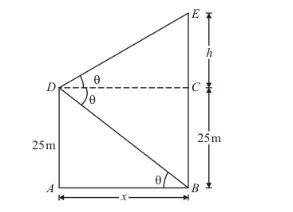# From the top of a cliff 25 m high the angle of elevation of a tower

Question:

From the top of a cliff 25 m high the angle of elevation of a tower is found to be equal to the angle of depression of the foot of the tower. The height of the tower is

(a) 25 m

(b) 50 m

(c) 75 m

(d) 100 m

Solution:

Given that: height of cliff ism and angle of elevation of the tower is equal to angle of depression of foot of the tower that is.

Now, the given situation can be represented as,Here, D is the top of cliff and BE is the tower.

Let $C E=h, A B=x$. Then, $A B=D C=x$

Here, we have to find the height of the tower BE.

So, we use trigonometric ratios.

In a triangle ABD,

$\Rightarrow \tan \theta=\frac{A D}{A B}$

$\Rightarrow \tan \theta=\frac{25}{x}$..............(1)

Again in a triangle,

$\tan \theta=\frac{C E}{C D}$

$\Rightarrow \tan \theta=\frac{h}{x}$

$\Rightarrow \frac{25}{x}=\frac{h}{x}$ $[$ Using (1) $]$

$\Rightarrow h=25$

Thus, height of the tower = BE = BC + CE = (25 + 25) m = 50 m

Hence, the correct option is.